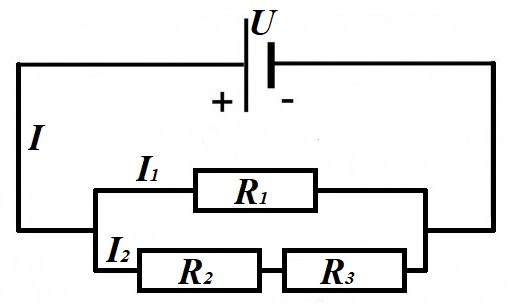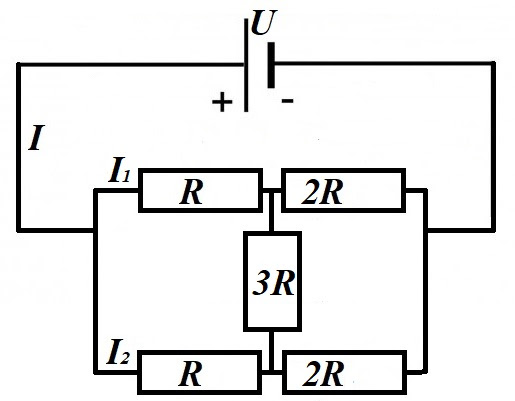## Saturday, April 4, 2020

### Unizor - Physics4Teens - Electromagnetism - DC Ohm's Law - Problems 1

Notes to a video lecture on http://www.unizor.com

Direct Current - Ohm's Law -
Problems 1

Problem A
Determine currents II1 and I2, if voltage at the source of electric power U and resistances R1R2 and R3 are given.Solution
R23 = R2 + R3
1/R = 1/R1 + 1/R23
I = U/R
I1 = U/R1
I2 = U/R23

Problem B
Consider a circuit that contains the source of electric field with difference of electric potential on its terminals (voltageU and a resistor with resistance R. The electric field forces the electrons to flow through the circuit, which means the field performs some work.
Express the work performed by an electric field during time t and its power in terms of voltage U, current I and resistance R.

Solution
Assume that q coulombs of electricity flows through a circuit during time t.
Using the definition of the difference of potentials (voltageU and the definition of the electric current I=q/t, the work performed by a field A equals to
A = U·q = U·I·t
and from the Ohm's Law I=U/R or U=I·R, the latter can be written as
A = I²·R·t = U²·t/R
From the definition of power as work performed in a unit of time W=A/t follows
W = I²·R = U²/R

Problem C
The Energy Conservation Law dictates that the work performed by an electric field to move electrons around a circuit defined by voltage Uamperage I and resistance R should result in something tangible.
This tangible result is the heat. The molecules inside the resistor are pushed around by electrons circulated in the circuit and, as a result, the temperature of the resistor increases.
The amount of heat produced in the resistor equals to amount of work performed by the electric field (Joule-Lenz Law).
Consider the circuit with a resistor being a regular incandescent lamp with a spiral made of tungsten with mass m=0.001kg.
The spiral resistance is R=200Ω.
The voltage produced by a source of electric field is 110V.
Tungsten specific heat capacity is c=134J/(kg·K).
We switch on the electricity in a circuit for the period of t=2sec.
What will be the rise in temperature of the tungsten spiral at the end?

Solution
Work performed by an electric field:
A = U²·t/R
(same as amount of heat inside the resistor)
The amount of heat is
Q = 110²·2/200 = 121 joules
Heat Q, mass m, change of temperature ΔT and specific heat c are connected by the following law:
Q = c·m·ΔT
Therefore,
ΔT = Q / (c·m)
Using the values given,
ΔT = 121/(134·0.001) = 903°K
This will be the rise of temperature after 2 sec of working electricity.

Problem D
Determine currents II1 and I2, if voltage at the source of electric power U and resistance R are given.Solution
The problem with this circuit is that it cannot be considered as consisting from parallel and series resistors. The resistor 3R, connecting top and bottom branches of a circuit, prevents it.
However, notice that the circuit is symmetrical, its top part (resistors R and 2R) is the same as the bottom part. From this symmetry we conclude that
I1 = I2 = I/2
and the voltage drops on resistors with resistance R on the top and on the bottom are the same
ΔU = (I/2)·R
Therefore, the difference in electric potentials at the ends of resistor 3R is zero and there is no electric current going through it, and it can be removed and excluded from all the calculations.
What's left after we exclude resistor 3R from consideration is parallel connection of two pairs of resistors connected in a series.
Total resistance of each branch (top or bottom) is a sum of two resistors R and 2R connected in a series
Rbranch = R + 2R = 3R
Total resistance of two branches together is calculated from a formula for parallel connection of two resistors with resistance 3R each
1/Rtotal = 1/(3R) + 1/(3R)
Rtotal = 3R/2
Now we can calculated the electric current I from the Ohm's Law
I = U/Rtotal = 2U/(3R)
I1 = I2 = I/2 = U/(3R)